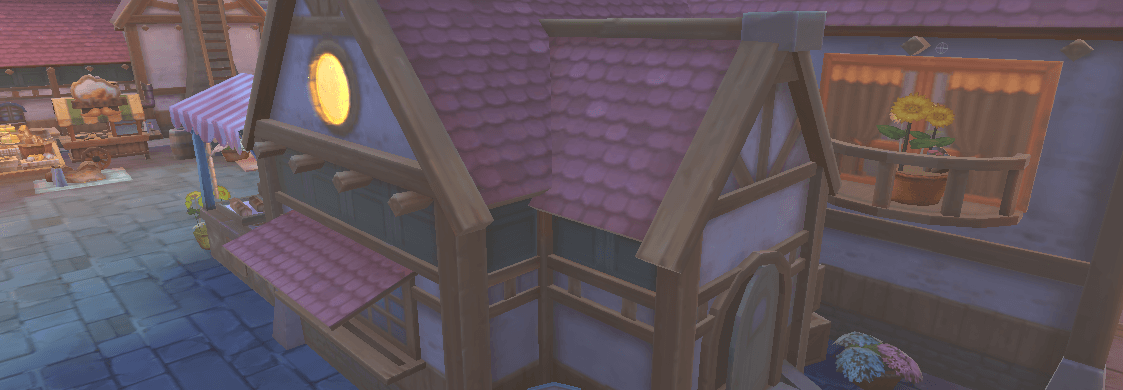# Unity Gamma校正转为线性空间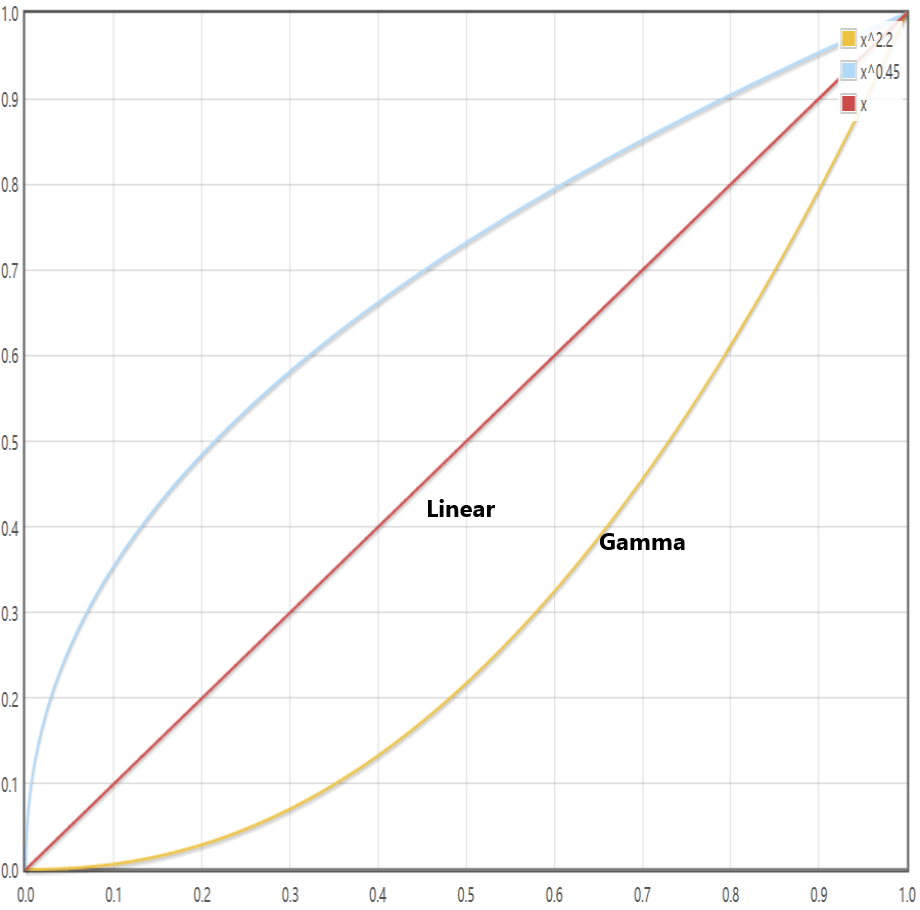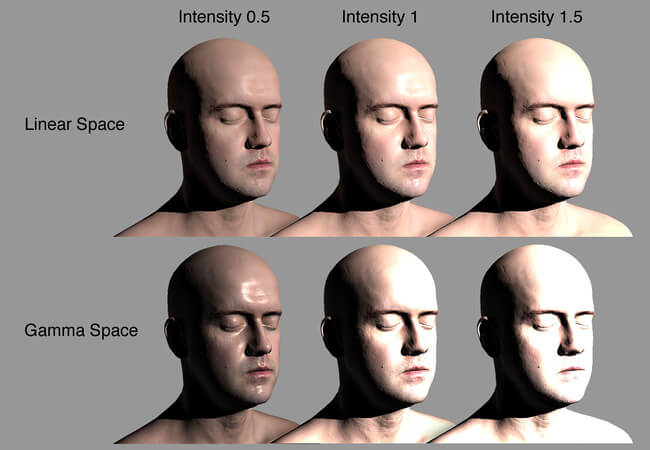Infinite 3D Head Scan by Lee Perry-Smith, licensed under a Creative Commons Attribution 3.0 Unported License (available from www.ir-ltd.net)

``````inline float GammaToLinearSpaceExact (float value)
{
if (value <= 0.04045F)
return value / 12.92F;
else if (value < 1.0F)
return pow((value + 0.055F)/1.055F, 2.4F);
else
return pow(value, 2.2F);
}
``````
``````inline half3 GammaToLinearSpace (half3 sRGB)
{
// Approximate version from http://chilliant.blogspot.com.au/2012/08/srgb-approximations-for-hlsl.html?m=1
return sRGB * (sRGB * (sRGB * 0.305306011h + 0.682171111h) + 0.012522878h);

// Precise version, useful for debugging.
//return half3(GammaToLinearSpaceExact(sRGB.r), GammaToLinearSpaceExact(sRGB.g), GammaToLinearSpaceExact(sRGB.b));
}
``````
`````` inline float LinearToGammaSpaceExact (float value)
{
if (value <= 0.0F)
return 0.0F;
else if (value <= 0.0031308F)
return 12.92F * value;
else if (value < 1.0F)
return 1.055F * pow(value, 0.4166667F) - 0.055F;
else
return pow(value, 0.45454545F);
}
``````
``````inline half3 LinearToGammaSpace (half3 linRGB)
{
linRGB = max(linRGB, half3(0.h, 0.h, 0.h));
// An almost-perfect approximation from http://chilliant.blogspot.com.au/2012/08/srgb-approximations-for-hlsl.html?m=1
return max(1.055h * pow(linRGB, 0.416666667h) - 0.055h, 0.h);

// Exact version, useful for debugging.
//return half3(LinearToGammaSpaceExact(linRGB.r), LinearToGammaSpaceExact(linRGB.g), LinearToGammaSpaceExact(linRGB.b))
}
``````

`````` half4 color = UNITY_SAMPLE_TEX2D(_MainTex, i.uv);
color.rgb = GammaToLinearSpace(color.rgb); // 转换到Linear Space
bakeGI += DecodeLightmap(UNITY_SAMPLE_TEX2D(unity_Lightmap, i.ambientOrLightmapUV.xy)).rgb; // 不要忽略预烘焙的Light Map

// 中间省略一些计算过程

colLit += color.rgb * bakeGI;
color.rgb = colLit;

color.rgb = LinearToGammaSpace(color.rgb * unity_ColorSpaceDouble); //转回Gamma Space
return color;
``````

“与此相关的有，一个Unity提供的与色彩空间相关的值，Unity_ColorSpaceDouble。这个值在Gamma Color Space时为2，在Linear Color Space时为4.594（2的2.2次方）。对于这个值可以这样来理解。一般在Gamma Color Space中将两个Color值相乘后，为了避免颜色变得很暗，会在后面乘以2。”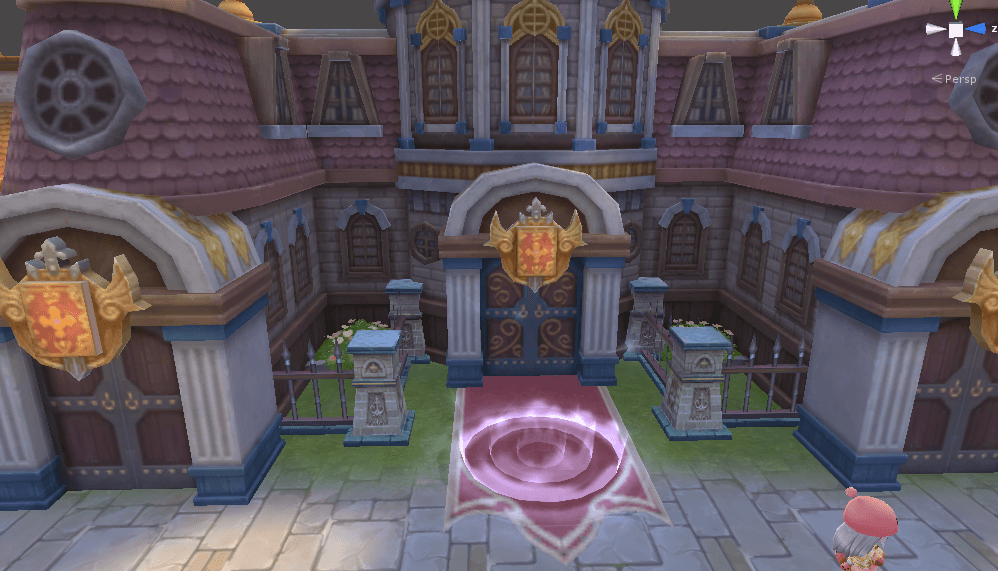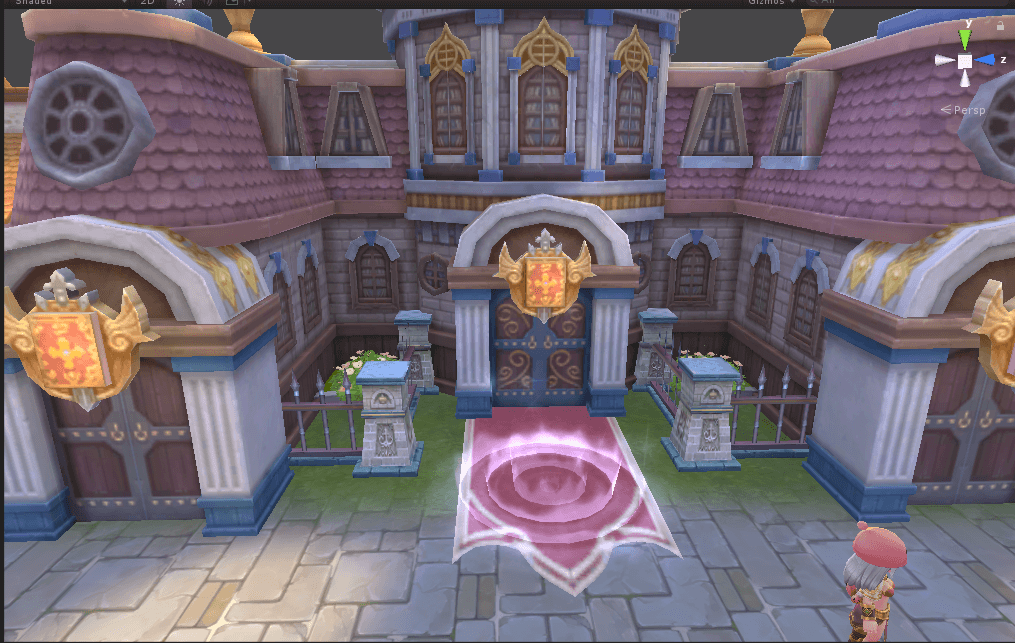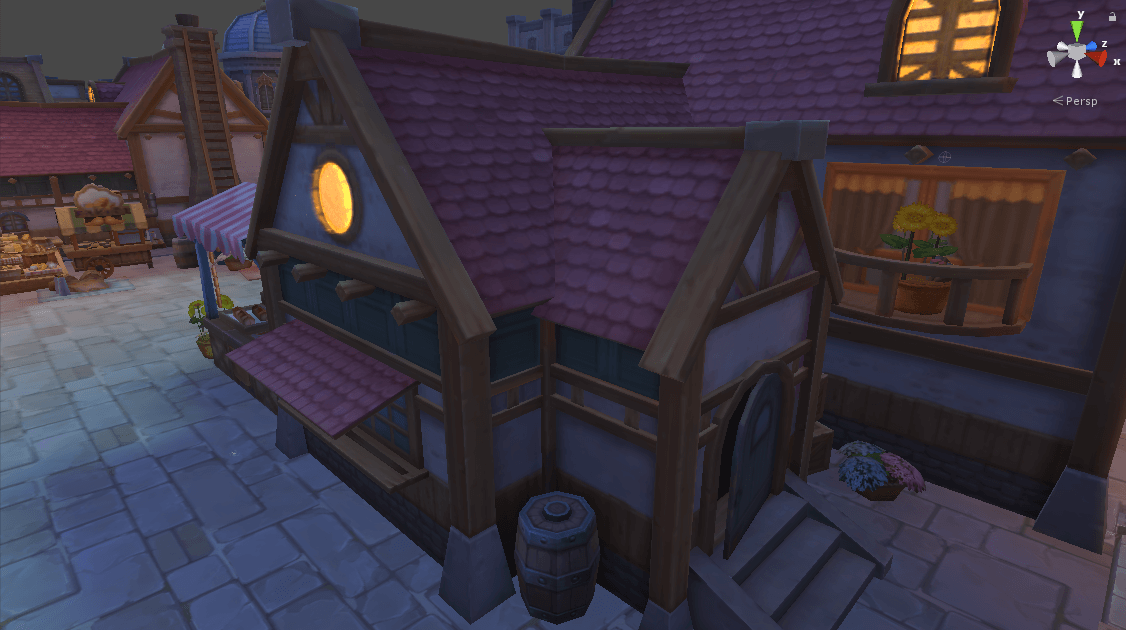QQ群：793972859